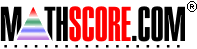Math Practice Online > free > lessons > Florida > 6th grade > Commutative Property 2

## Commutative Property 2

Completion of equations that demonstrate the commutative property. For example, since 3*5=15, 5*3=15.

 Sample Problems for Commutative Property 2 Lesson for Commutative Property 2

### This topic aligns to the following state standards

Grade 3: Num      d. applying the commutative property of multiplication, such as 7 x 3 = 3 x 7
Grade 4: Num      c. the commutative, associative, and distributive properties of multiplication.
Grade 5: Num 5. Explains and demonstrates the commutative, associative, and distributive properties of multiplication.
Grade 6: Num 3. Knows and applies the commutative, associative, and distributive properties in the addition and multiplication of rational numbers.
Grade 7: Num 3. Applies the properties of rational numbers to solve problems (commutative, associative, distributive, identity, equality, inverse).
Grade 8: Num 3. Applies the properties of real numbers to solve problems (commutative, associative, distributive, identity, equality, inverse, and closure).

Copyright Accurate Learning Systems Corporation 2008.